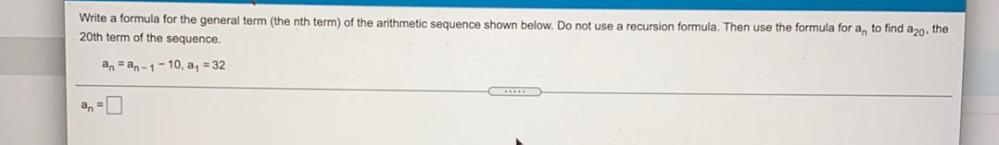Question:

# Write a formula for the general term (the nth term) of the arithmetic sequence shown below. Do not use 20th term of the sequenceWrite a formula for the general term (the nth term) of the arithmetic sequence shown below. Do not use 20th term of the sequence. recursion formula. Then use the formula for a, to find azo, the anan-1-10, a, = 32 RED an-